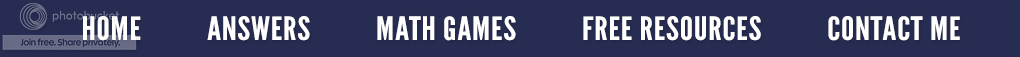### Fibonacci Numbers and The Golden RatioFibonacci
Even if you were taught about the Fibonacci number sequence in school, you probably don’t remember much about it. As with other higher levels of math, many aren’t sure how Fibonacci could possibly be relevant to their real lives; so, why should they even attempt to remember him or his sequence? In reality, Fibonacci numbers are something you come across practically every day. Even so, let’s go back and start at the beginning.

The Fibonacci number sequence is named after Leonardo of Pisa (1175-1240), who was known as Fibonacci. (I love to say that name because it sounds like I know a foreign language.) In mathematics, Fibonacci numbers are this sequence of numbers:
As you can see, it is a pattern, (all math is based on patterns). Can you figure out the number that follows 89? Okay, let's pretend I waited for at least 60 seconds before giving you the answer….144. By definition, the first two numbers in the Fibonacci sequence are 0 and 1, and each subsequent number is the sum of the previous two. For those who are still having difficulty (like my daughter who is sitting here), it is like this.

The next number is found by adding up the two numbers that precede it.
• The 8 is found by adding the two numbers before it (3 + 5)
• Similarly, 13 is found by adding the two numbers before it (5 + 8),
• And the 21 is (8 + 13), and so on!
It is that simple! For those who just love patterns, here is a longer list:

Can you figure out the next few numbers?

The Fibonacci sequence can be written as a "Rule “which is:   xn = xn-1 + xn-2   The terms are numbered from 0 forwards as seen in the chart below.   xn is the term number n.   xn-1 is the previous term (n-1) and xn-2 is the term before that (n-2)
﻿Sometimes scientists and mathematicians enjoy studying patterns and relationships because they are interesting, but frequently it's because they help to solve practical problems. Number patterns are regularly studied in connection to the world we live in so we can better understand it. As mathematical connections are uncovered, math ideas are developed to help us be aware of the relationship between math and the natural world.

As stated previously, we come across Fibonacci numbers almost every day in real life. For instance, my husband and I were at the Wonders of Wildlife Aquarium in Springfield, Missouri. (If you haven't been, you should go because it is spectacular.) He was noticing how the herrings were swimming counter clockwise and discussing the Coriolis effect with the guide. When we got to the lower levels, where the sharks were, they were all swimming in a counterclockwise direction as well. I asked my rocket scientist husband why this was and again he said, with a straight face, "The Coriolis Effect."Inside of a Nautilus Shell
I then spied seashells and started talking about Fibonacci numbers and the Golden Ratio. (I know the visitors around us were wondering just who we were!) On the right, you will see a picture of the inside of a Nautilus Shell taken by me! It clearly shows the Golden Ratio. (The Golden Ratio is a special number equal to about 1.6180339887498948482. The Greek letter Phi is used to refer to this ratio. Like Pi, the digits of the Golden Ratio go on forever without repeating.) Many shells, including snail shells and nautilus shells, are perfect examples of the Golden spiral.Are you still not sure what I am talking about? Have you ever watched the Disney movie entitled Donald in Mathmagic Land? (It's an old one thatThe Golden Ratio
you can find on You Tube.) Well, in the movie they talk about the Golden ratio. This is a proportion that is found in nature and in architecture. The proportion creates beauty. And that proportion is the Fibonacci sequence! If you divide consecutive Fibonacci numbers you will always get the Golden ratio. Try it! Start with the big numbers. If you divide 89 by 55, you get 1.61. If you divide 55 by 34, you get 1.61. If you divide 34 by 21, you get 1.61, and so on. You can look up the Golden Ratio and explore it more. It’s fun!

As I close, here are four questions to think about:
1. How might knowing this number pattern be useful?
2. What kinds of things can the numbers in the Fibonacci sequence represent?
3. Where is the Golden Ratio found in the human body?
4. Why is the golden rectangle important in architecture and art?